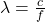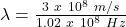Question

The FM station broadcasts traditional music at 102 MHz on your radio units for FM frequencies are given in MHz. Find the wave length of radio station in metres (m), nanometre(nm) and angstrom(Å).

in progress 0
5 months 2021-08-28T11:21:40+00:00 1 Answers 0 views 0

λ = 2.94 m (in meters)

λ = 2.94 x 10⁹ nm (in nanometers)

λ = 2.94 x 10¹⁰ A (in angstrom)

Explanation:

The wavelength of the radio station can be given as follows:where,

λ = wavelength = ?

f = frequency = 102 MHz = 1.02 x 10⁸ Hz

c = speed of light = 3 x 10⁸ m/s

Therefore,λ = 2.94 m (in meters)

λ = (2.94 m)(10⁹nm / 1 m)

λ = 2.94 x 10⁹ nm (in nanometers)

λ = (2.94 m)(10¹⁰ A / 1 m)

λ = 2.94 x 10¹⁰ A (in angstrom)